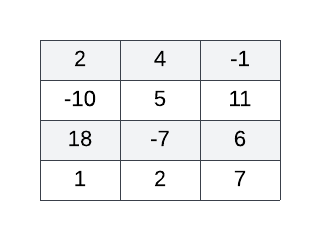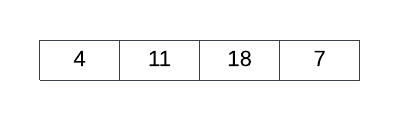Login or Create an Account to view the mark scheme, comment, and add to a test
Given a 2D array called ARR of size 4x3 and an empty 1D array called BIGGEST of size 4, construct an algorithm to sequentially fill BIGGEST with the biggest number in each ARR row such that the index of each number in BIGGEST is the same as its original ARR row index. The more positive or less negative a number, the bigger it is - for example, 7, 0 and -2 are all considered bigger than -10. ARR may contain one or more negative numbers. For example, consider the following 2D array:The correctly functioning algorithm applied to the 2D array above yields the following 1D array:HomeThe Right Test - Fast